Courses

# Previous year Questions (2016-20) - Quadratic Equations and Inequalities (Inequalities) Notes | EduRev

## Commerce : Previous year Questions (2016-20) - Quadratic Equations and Inequalities (Inequalities) Notes | EduRev

The document Previous year Questions (2016-20) - Quadratic Equations and Inequalities (Inequalities) Notes | EduRev is a part of the Commerce Course Mathematics (Maths) Class 11.
All you need of Commerce at this link: Commerce

Q.1. Let α and β be two real roots of the equation (k + 1)tan2x  - √2 λ tan x =  (1-k), where k (≠ - 1) and λ are real numbers. If 2 tan2(α + β) = 50, then a value of λ is    (2020)
(1) 10√2
(2) 10
(3) 5
(4) 5√2
Ans.
(2)
Solution. The given quadratic equation is
(k + 1)tan2x - √2λ tan x + (k - 1) = 0
Given that α and β be the two real roots of the given equation, then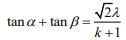...(1)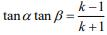...(2)
Now,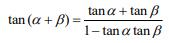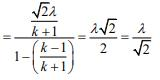Now, tan2(α + β) = 50 ⇒ λ2/2  = 50
⇒ λ= 100 ⇒ λ = ±10

Q.2. Let α and β be the roots of the equation x2 - x - 1 = 0. If pk = (α)k + (β)k, k ≥ 1, then which one of the following statements is not true?    (2020)
(1) p3 = p5 - p4
(2) p5 = 11
(3) (p1 + p2 + p3 + p4 + p5) = 26
(4) p5 = p2.p3
Ans.
(4)
Solution. Given, α and β be the roots of the equation x2 - x - 1 = 0. Therefore,
α + β = 1 and αβ = -1
Now, pk = (α)+ (β)k
p1 = α + β  = 1 ...(1)
p2 = α2 + β2 = (α + β)2 - 2αβ
⇒ p2 = 1 + 2 = 3 ...(2)
p3 = α3 + β3 = (α + β)3 - 3αβ(α + β)
p3 = 1 + 3 = 4 ...(3)
Since α and β be the roots of the equation x2 - x − 1 = 0, then
α2 - α - 1 = 0 ⇒ αk - αk-1 - αk-2 = 0 ...(4)
β2 - β - 1 = 0 ⇒ βk - βk-1 - βk-2 = 0 ...(5)
From Eqs. (4) and 5, we get pk = pk-1 + pk-2. Hence, p4 = 7 and p5 = 11 ⇒ p5 ≠ p2.p3

Q.3. If the equation x2 + bx + 45 = 0 (b ∈ R) has conjugate complex roots and they satisfy |z+1| = 2√10, then    (2020)
(1) b2 - b = 30
(2) b+ b = 72
(3) b2 - b = 42
(4) b2 + b = 12
Ans.
(1)
Solution. Let z = α ± iβ be the roots of the x2 + bx + 45 = 0, then
α + iβ + α - iβ = -b ⇒ 2α = -b ...(1)
(α + iβ)(α - iβ) = 45 ⇒ (α2 + β2) = 45 ...(2)
The roots satisfy the equation |z + 1| = 2√10, then
(α + 1)2 + β2 = 40 ...(3)
From Eqs. (2) and (3), we get
(α + 1)2 - α2 = -5 ⇒ 2α + 1 = -5 ⇒ α = -3
Hence, b2 + b = (-6)2 - 6 = 30

Q.4. The least positive value of ‘a’ for which the equation 2x+(a-10)x+ 33/2 = 2a has real roots is _____.    (2020)
Ans.
(8.00)
Solution. The equation 2x+(a-10)x + 33/2 = 2a has real roots, therefore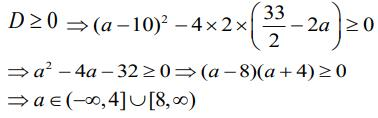Hence, the least positive value of ‘a’ is 8.

Q.5. Let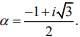If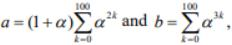then a and b are the roots of the quadratic equation    (2020)
(1) x2 + 101x + 100 = 0
(2) x2 - 102x + 101 = 0
(3) x2 - 101x + 100 = 0
(4) x2 + 102x + 101 = 0
Ans.
(2)
Solution. Given,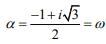Now,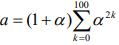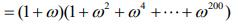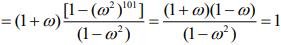(since ω3 = 1)
b = 1 + ω+ ω6 + ... + ω300 = 101
Hence, the equation of quadratic equation is
x2 - (a + b)x + ab = 0 ⇒ x2 - 102x  + 101 = 0

Q.6. Let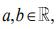a ≠ 0 be such that the equation ax2 - 2bx  + 5 = 0 has a repeated root α, which is also a root of the equation x2 - 2bx - 10 = 0. If β  is the other root of this equation, then α2 + β2 is equal to    (2020)
(1) 25
(2) 26
(3) 28
(4) 24
Ans.
(1)
Solution. The equation ax2 - 2bx + 5 = 0 has a repeated root α, then
D = 0 ⇒ 4b2 - 4 . a . 5 = 0 ⇒ b2 = 5a ...(1)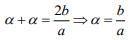...(2)
Since α is also, the root of equation x2 - 2bx - 10 = 0. So,
α2 - 2bα - 10 = 0 ...(3)
2 - 2bα + 5 = 0 ...(4)
From Eqs. (3) and (4), we get
(a - 1)α2 + 15 = 0 ⇒ (a - 1)b2 + 15a2 = 0
⇒ (a - 1) x 5a +15a2 = 0 ⇒ 20a2 - 5a = 0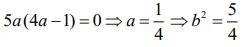Now, α + β = 2b and αβ = -10
Hence, (α + β)2 = α2 + β+ 2αβ = 4b2
⇒α2 + β= 5 - 2(-10) = 25

Q.7. Let α and β be two roots of the equation x2 + 2x + 2 = 0, then α1515 is equal to:   (2019)
(1) -256
(2) 512
(3) -512
(4) 256
Ans.
(1)
Solution. Consider the equation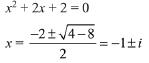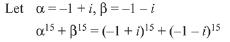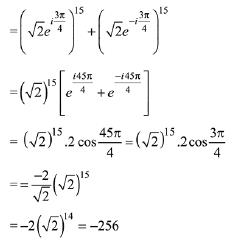Q.8. If both the roots of the quadratic equation x2 - mx + 4 = 0 are real and distinct and they lie in the interval [1,5], then m lies in the interval:    (2019)
(1) (-5,-4)
(2) (4,5)
(3) (5,6)
(4) (3,4)
Ans.
(2)
Solution. Given quadratic equation is: x2 -mx + 4 = 0 Both the roots are real and distinct.
So, discriminant b2 - 4ac > 0.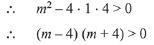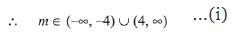Since, both roots lies in [1, 5]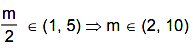....(ii)
And 1 · (1 - m + 4) > 0 ⇒ m < 5
∴ m ∈(∞ - 5)   ...(iii)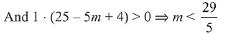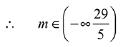...(iv)
From (i), (ii), (iii) and (iv), m ∈ (4, 5)

Q.9. The number of all possible positive integral values of α for which the roots of the quadratic equation, 6x2 - 11x + α = 0 are rational numbers is:    (2019)
(1) 3
(2) 2
(3) 4
(4) 5
Ans.
(1)
Solution. The roots of 6x2 - 11x + α = 0 are rational numbers.
∴ Discriminant D must be perfect square number.
D = (-11)2 - 4 · 6 · α
= 121- 24α    must be a perfect square
Hence, possible values for α are
α = 3, 4, 5.
∴ 3 positive integral values are possible.

Q.10. If 5, 5r, 5r2 are the lengths of the sides of a triangle, then r cannot be equal to:    (2019)
(1) 3/4
(2) 5/4
(3) 7/4
(4) 3/2
Ans.
(3)
Solution.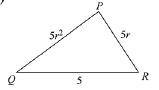ΔPQR is possible if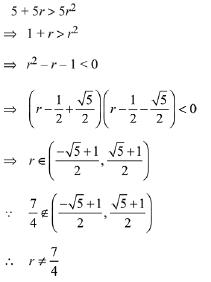Q.11. Consider the quadratic equation (c - 5)x2 - 2cx + (c - 4) = 0, c ≠ 5. Let S be the set of all integral values of c for which one root of the equation lies in the interval (0, 2) and its other root lies in the interval (2, 3). Then the number of elements in S is:    (2019)
(1) 18
(2) 12
(3) 10
(4) 11
Ans.
(4)
(c - 5) x2 - 2cx + (c - 4) = 0
Now,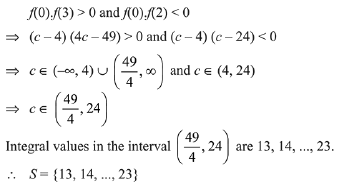Q.12. The value of λ such that sum of the squares of the roots of the quadratic equation, x2 + (3 - λ)x + 2 = λ has the least value is:    (2019)
(1) 15/8
(2) 1
(3) 4/9
(4) 2
Ans.
(4)
Solution. The given quadratic equation is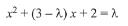Sum of roots =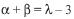Product of roots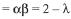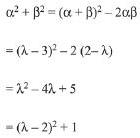For α2to be minimum, λ=2.

Q.13. If one real root of the quadratic equation 81x2 + kx + 256 = 0 is cube of the other root, then a value of k is :    (2019)
(1) -81
(2) 100
(3) 144
(4) -300
Ans.
(4)
Solution. Let α and β be the roots of the equation.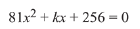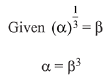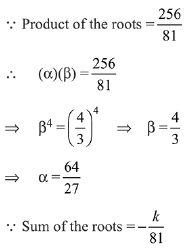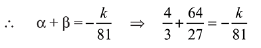k = -300

Q.14. If α,β be the ratio of the roots of the quadratic equation in x, 3m2x2 + m(m - 4)x + 2 = 0, then the least value of m for which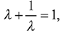is:    (2019)
(1) 2-√3
(2) 4-3√2
(3) -2 + √2
(4) 4-2√3
Ans.
(2)
Solution. Let roots of the quadratic equation are α, β.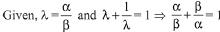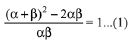The quadratic equation is, 3m2x2 + m(m - 4) x + 2 = 0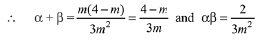Put these values in eq (1),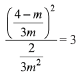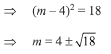Therefore, least value is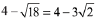Q.15. The number of integral values of m for which the quadratic expression, (1 + 2m)x2 -2(1 + 3m)x + 4(1 + m), x ∈ R, is always positive, is:    (2019)
(1) 3
(2) 8
(3) 7
(4) 6
Ans.
(3)
Solution. Let the given quadratic expression
(1 + 2m)x2 - 2(1 + 3m)x + 4(1 + m), is positive for all x ∈ R, then
1 + 2m > 0   ...(1)
D<0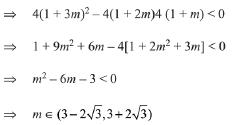From (1)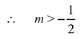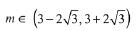Then, integral values of m = {0, 1,2, 3,4, 5, 6}
Hence, number of integral values of m = 7

Q.16. If α and β be the roots of the equation x2 - 2x + 2 = 0, then the least value of n for which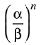= 1 is:    (2019)
(1) 2
(2) 5
(3) 4
(4) 3
Ans.
(3)
Solution. The given quadratic equation is x2 - 2x + 2 = 0
Then, the roots of the this equation are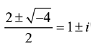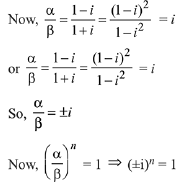⇒ n must be a multiple of 4.
Hence, the required least value of n = 4.

Q.17. The sum of the solutions of the equation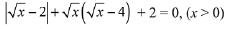is equal to:    (2019)
(1) 9
(2) 12
(3) 4
(4) 10
Ans.
(4)
Solution.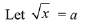∴ given equation will become: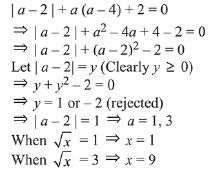Hence, the required sum of solutions of the equation = 10

Q.18. The number of integral values of m for which the equation (1 + m2)x2 - 2(1 + 3m)x + (1 + 8m) = 0 has no real root is:    (2019)
(1) 1
(2) 2
(3) infinitely many
(4) 3
Ans.
(3)
Solution. Given equation is∵ equation has no real solution
D< 0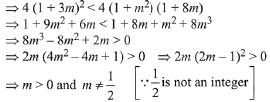⇒ number of integral values of m are infinitely many.

Q.19. Let p, q ∈ R. If 2 - √3 is a root of the quadratic equation, x2 + px + q = 0, then:    (2019)
(1) p2-4q + 12 = 0
(2) q2 - 4p - 16 = 0
(3) q2 + 4p + 14 = 0
(4) p2 - 4q - 12 = 0
Ans.
(4)
Solution. Since 2 - √3 is a root of the quadratic equation x2 + px + q = 0
∴ 2 + √3 is the other root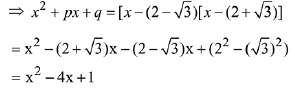Now, by comparing p = -4, q = 1
⇒ p- 4q - 12= 16 - 4 - 12 = 0

Q.20. If m is chosen in the quadratic equation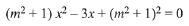such that the sum of its roots is greatest, then the absolute difference of the cubes of its roots is:    (2019)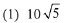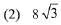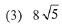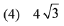Ans.
(3)
Solution.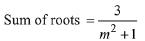∵ sum of roots is greatest ∴ m = 0
Hence equation becomes x2 - 3x + 1 = 0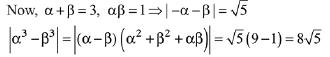Q.21. If α and β are the roots of the quadratic equation, x2 + x sinθ - 2sinθ = 0 0,θ ∈ (0, π/2), then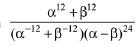is equal to:    (2019)
(1)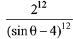(2)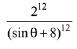(3)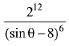(4)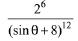Ans.
(2)
Solution. Given equation is, x2 + x sinθ - 2 sinθ = 0
α + β = - sinθ and αβ = - 2 sinθ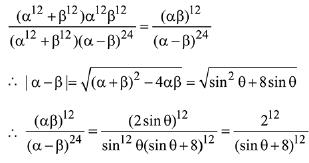Q.22. The number of real roots of the equation 5 + |2x -1| = 2x (2x - 2) is:    (2019)
(1) 3
(2) 2
(3) 4
(4) 1
Ans.
(4)
Solution.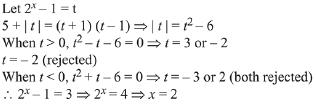Q.23. Let S = {x ∈ R : x ≥ 0 and 2 |√x - 3| + √x (√x - 6) + 6 = 0}. Then S:   (2018)
(1) is an empty set
(2) contains exactly one element
(3) contains exactly two element
(4) contains exactly four elements
Ans.
(3)
Solution.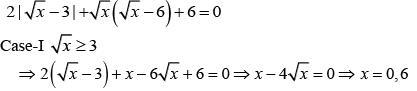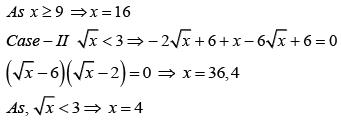There are exactly two elements in the given set.

Q.24. If λ ϵ R is such that the sum of the cubes of the roots of the equation, x2 + (2 - λ)x + (10 - λ) = 0 is minimum, then the magnitude of the difference of the roots of this equation is:    (2018)
(1) 2√5
(2) 20
(3) 2√7
(4) 4√7
Ans.
(1)
Solution.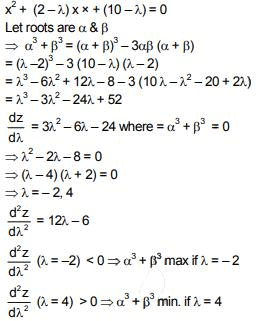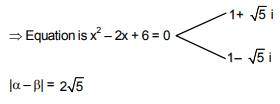Q.25. Let p, q and r be real numbers (p ≠ q, r ≠ 0), such that the roots of the equation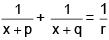are equal in magnitude but opposite in sign, then the sum of squares of these roots is equal to:    (2018)
(1) p2 + q2
(2)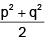(3) 2(p2 + q2)
(4) p2 + q2 + r
Ans.
(1)
Solution. (2x + p + q) r = (x + p) (x + q)
x2 + (p + q - 2r) x + pq - pr - qr = 0
p + q = 2r    …….. (i)
α2 + β2 = (α + β)2 - 2αβ
= 0 - 2 [pq - pr - qr]  = - 2pq + 2r (p + q)  = - 2pq + (p + q)2 = p2 + q2

Q.26. The number of values of k for which the system of linear equations,
(k + 2)x + 10y = k
kx + (k + 3) y = k - 1 has no solution, is:    (2018)
(1) 1
(2) 2
(3) 3
(4) 4
Ans.
(1)
Solution. For no solution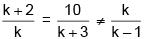(k + 2) (k + 3) = 10 k
k2 - 5k + 6 = 0
⇒ k = 2,3
k ≠ 2  for k = 2 both lines identical
so k = 3 only
so number of values of k is 1

Q.27. If, for a positive integer n, the quadratic equation, x(x + 1) + (x + 1) (x + 2) + ..... + (x + n - 1) (x + n) = 10n has two consecutive integral solutions, then n is equal to    (2017)
(1) 11
(2) 12
(3) 9
(4) 10
Ans.
(1)
Solution.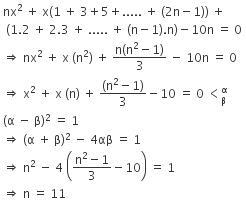Q.28. Let p(x) be a quadratic polynomial such that p(0) = 1. If p(x) leaves remainder 4 when divided by x – 1 and it leaves remainder 6 when divided by x + 1, then:    (2017)
(1) p(–2) = 19
(2) p(2) = 19
(3) p(–2) = 11
(4) p(2) = 11
Ans.
(1)
Solution. p(x) = ax2 + bx + c
p(0) = 1 = c = 1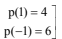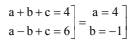p(x) = 4x2 – x + 1
p(–2) = 16 + 2 + 1 = 19

Q.29. The sum of all the real values of x satisfying the equation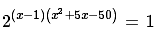is:    (2017)
(1) 16
(2) –5
(3) –4
(4) 14
Ans.
(3)
Solution. (x – 1) (x2 + 5x – 50) = 0
⇒ (x – 1) (x + 10) (x – 5) = 0
⇒ x = 1, 5, –10
Therefore, sum = –4

Q.30. The sum of all real values of x satisfying the equation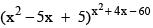is:    (2016)
(1) 3
(2) -4
(3) 6
(4) 5
Ans.
(1)
Solution.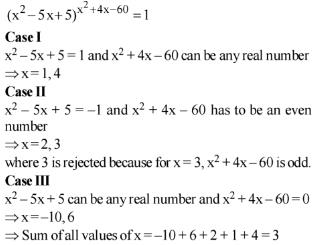Q.31. If the equations x2 + bx – 1 = 0 and x2 + x + b = 0 have a common root different from –1, then |b| is equal to    (2016)
(1) √2
(2) 2
(3) √3
(4) 3
Ans.
(3)
Solution. x2 + bx – 1 = 0 & x2 + x + b = 0 have common root α.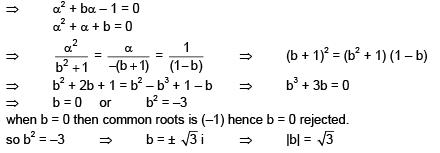Q.32. If x is a solution of the equation,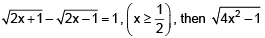is equal to    (2017)
(1) 2
(2) 3/4
(3) 2√2
(4) 1/2
Ans.
(2)
Solution.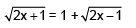Squaring on both sides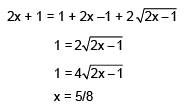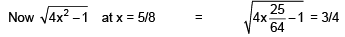,

,

,

,

,

,

,

,

,

,

,

,

,

,

,

,

,

,

,

,

,

;